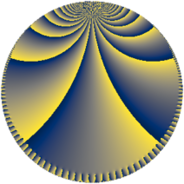# Properties

 Label 384.7.hLevel $384$ Weight $7$ Character orbit 384.h Rep. character $\chi_{384}(65,\cdot)$ Character field $\Q$ Dimension $96$ Newform subspaces $8$ Sturm bound $448$ Trace bound $3$

# Related objects

## Defining parameters

 Level: $$N$$ $$=$$ $$384 = 2^{7} \cdot 3$$ Weight: $$k$$ $$=$$ $$7$$ Character orbit: $$[\chi]$$ $$=$$ 384.h (of order $$2$$ and degree $$1$$) Character conductor: $$\operatorname{cond}(\chi)$$ $$=$$ $$24$$ Character field: $$\Q$$ Newform subspaces: $$8$$ Sturm bound: $$448$$ Trace bound: $$3$$ Distinguishing $$T_p$$: $$5$$, $$11$$

## Dimensions

The following table gives the dimensions of various subspaces of $$M_{7}(384, [\chi])$$.

Total New Old
Modular forms 400 96 304
Cusp forms 368 96 272
Eisenstein series 32 0 32

## Trace form

 $$96 q + O(q^{10})$$ $$96 q + 300000 q^{25} + 88160 q^{33} + 1201632 q^{49} - 488480 q^{57} + 1028160 q^{73} - 2388512 q^{81} + 2505216 q^{97} + O(q^{100})$$

## Decomposition of $$S_{7}^{\mathrm{new}}(384, [\chi])$$ into newform subspaces

Label Dim $A$ Field CM Traces $q$-expansion
$a_{2}$ $a_{3}$ $a_{5}$ $a_{7}$
384.7.h.a $2$ $88.341$ $$\Q(\sqrt{6})$$ $$\Q(\sqrt{-6})$$ $$0$$ $$-54$$ $$0$$ $$0$$ $$q-3^{3}q^{3}+7\beta q^{5}-17\beta q^{7}+3^{6}q^{9}+\cdots$$
384.7.h.b $2$ $88.341$ $$\Q(\sqrt{-2})$$ $$\Q(\sqrt{-2})$$ $$0$$ $$-46$$ $$0$$ $$0$$ $$q+(-23+\beta )q^{3}+(329-46\beta )q^{9}-2338q^{11}+\cdots$$
384.7.h.c $2$ $88.341$ $$\Q(\sqrt{-2})$$ $$\Q(\sqrt{-2})$$ $$0$$ $$46$$ $$0$$ $$0$$ $$q+(23-\beta )q^{3}+(329-46\beta )q^{9}+2338q^{11}+\cdots$$
384.7.h.d $2$ $88.341$ $$\Q(\sqrt{6})$$ $$\Q(\sqrt{-6})$$ $$0$$ $$54$$ $$0$$ $$0$$ $$q+3^{3}q^{3}+7\beta q^{5}+17\beta q^{7}+3^{6}q^{9}+\cdots$$
384.7.h.e $8$ $88.341$ $$\mathbb{Q}[x]/(x^{8} - \cdots)$$ None $$0$$ $$-72$$ $$0$$ $$0$$ $$q+(-9+\beta _{5})q^{3}-\beta _{6}q^{5}+(\beta _{3}+\beta _{6}+\cdots)q^{7}+\cdots$$
384.7.h.f $8$ $88.341$ $$\mathbb{Q}[x]/(x^{8} - \cdots)$$ None $$0$$ $$72$$ $$0$$ $$0$$ $$q+(9-\beta _{4})q^{3}-\beta _{6}q^{5}+(-\beta _{3}-\beta _{6}+\cdots)q^{7}+\cdots$$
384.7.h.g $24$ $88.341$ None $$0$$ $$0$$ $$0$$ $$0$$
384.7.h.h $48$ $88.341$ None $$0$$ $$0$$ $$0$$ $$0$$

## Decomposition of $$S_{7}^{\mathrm{old}}(384, [\chi])$$ into lower level spaces

$$S_{7}^{\mathrm{old}}(384, [\chi]) \cong$$ $$S_{7}^{\mathrm{new}}(24, [\chi])$$$$^{\oplus 5}$$$$\oplus$$$$S_{7}^{\mathrm{new}}(96, [\chi])$$$$^{\oplus 3}$$$$\oplus$$$$S_{7}^{\mathrm{new}}(192, [\chi])$$$$^{\oplus 2}$$